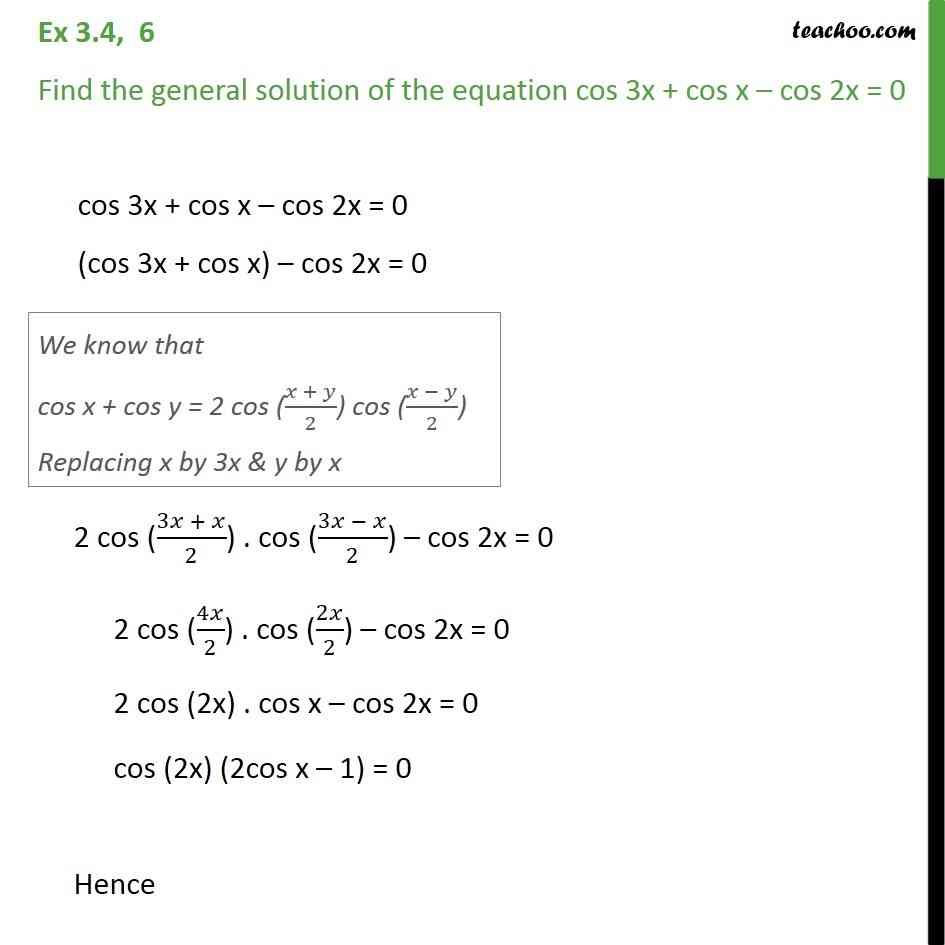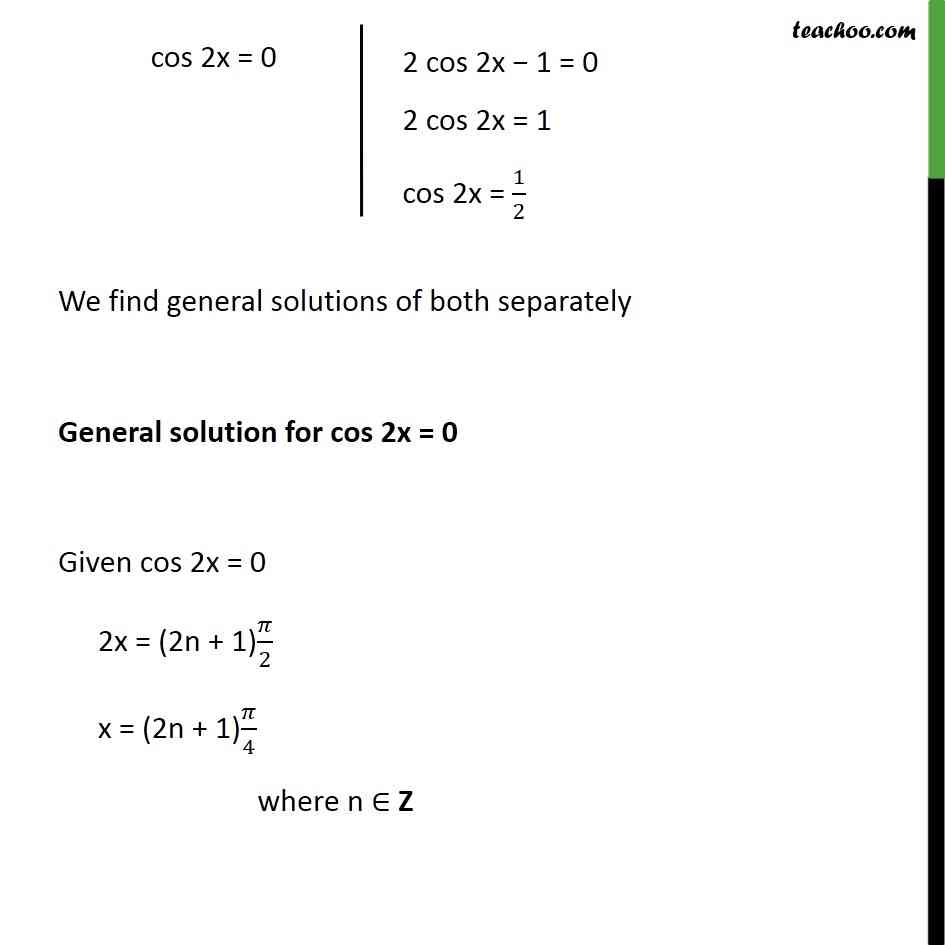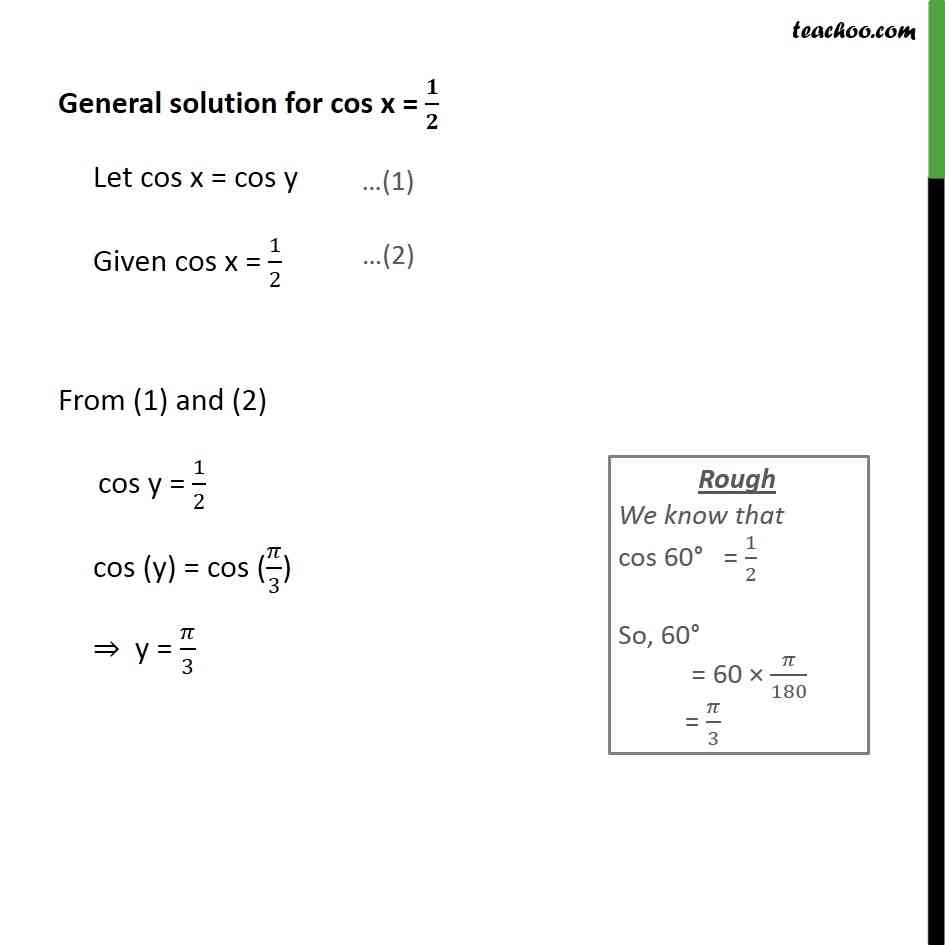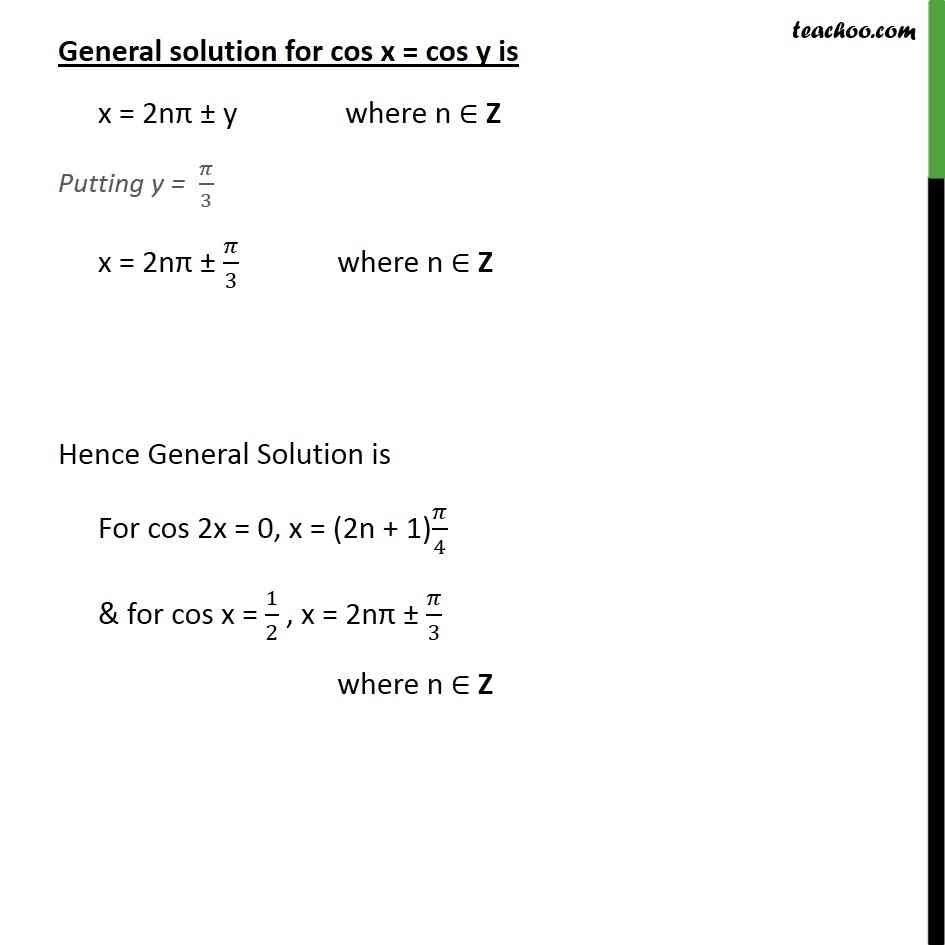1. Chapter 3 Class 11 Trigonometric Functions
2. Serial order wise
3. Ex 3.4

Transcript

Ex 3.4, 6 Find the general solution of the equation cos 3x + cos x – cos 2x = 0 cos 3x + cos x – cos 2x = 0 (cos 3x + cos x) – cos 2x = 0 2 cos ((3𝑥 + 𝑥)/2) . cos ((3𝑥 − 𝑥)/2) – cos 2x = 0 2 cos (4𝑥/2) . cos (2𝑥/2) – cos 2x = 0 2 cos (2x) . cos x – cos 2x = 0 cos (2x) (2cos x – 1) = 0 Hence cos 2x = 0 or (2cos x – 1) = 0 cos 2x = 0 or 2cos x = 1 cos 2x = 0 or cos x = 1/2 We find general solutions of both separately General solution for cos 2x = 0 Let cos x = cos y ⇒ cos 2x = cos 2y Given cos 2x = 0 From (1) and (2) cos 2y = 0 cos 2y = cos 90° cos 2y = cos 𝜋/2 2y = 𝜋/2 General solution for cos 2x = cos 2y is given by 2x = 2nπ ± 2y where n ∈ Z putting 2y = 𝜋/2 2x = 2nπ ± 𝜋/2 x = 1/2 (2nπ ± 𝜋/2) x = nπ ± 𝜋/2 General solution for cos x = 𝟏/𝟐 Let cos x = cos y Given cos x = 1/2 From (3) and (4) cos y = 1/2 cos (y) = cos (𝜋/3) ⇒ y = 𝜋/3 General solution for cos x = cos y is x = 2nπ ± y where n ∈ Z putting y = 𝜋/3 x = nπ ± 𝜋/3 where n ∈ Z Hence General Solution is For cos 2x = 0, x = nπ ± 𝜋/2 & for cos x = 1/2 , x = nπ ± 𝜋/3 where n ∈ Z

Ex 3.4